# 均线多头排列选股教程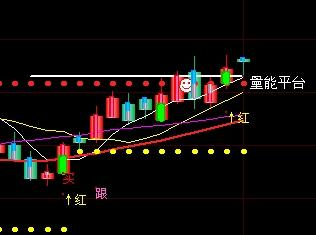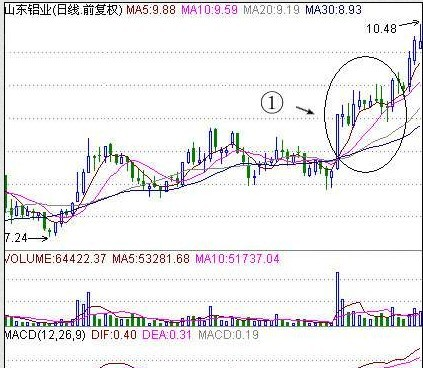“均线多头排列”选牛股（例：G中黄金、G中材）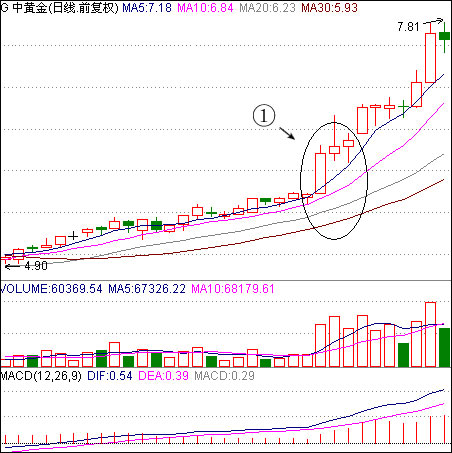1 部分医药,酒类,农林及部分小盘股为代表长庄慢牛股;跌的少,涨的也相对缓.偶尔也有拉长阳加速.002069是经典例子.
2 有色金属板块中以000960,002237为代表的45度稳步推升品种
3 先挖坑洗盘,突破中长期均线压力后开始阶段性加速上涨,启动主升浪行情,600086,600367为代表.
4 以稀土,锂,磁为代表的新兴产业板块,中长期上升趋势保持完好,均线形成完全多头排列后开始阶段性加速上涨,大角度上攻.核心代表600259.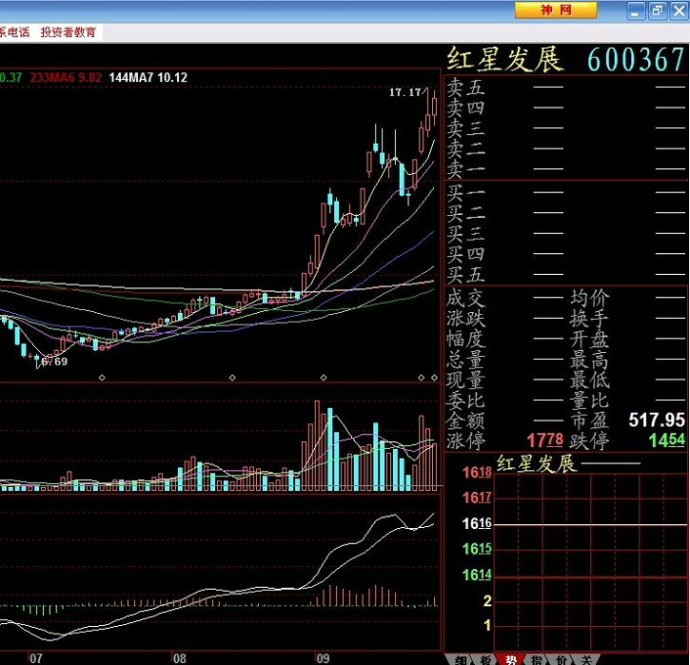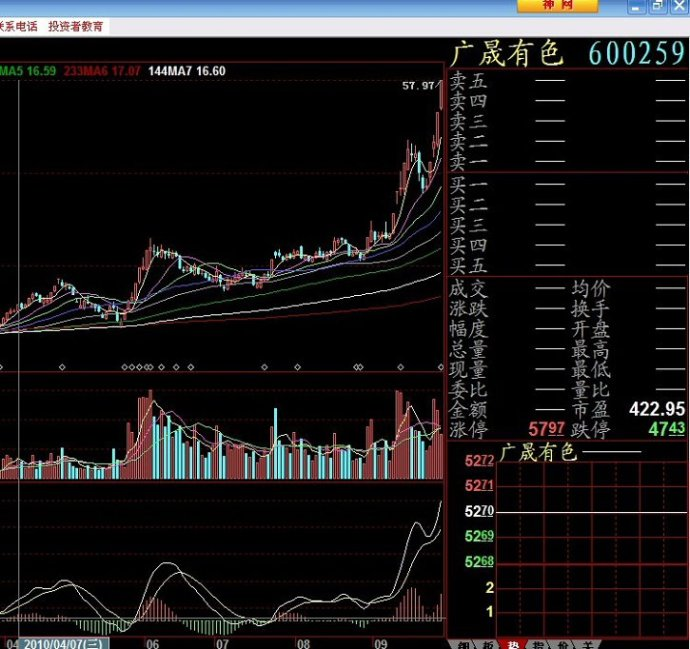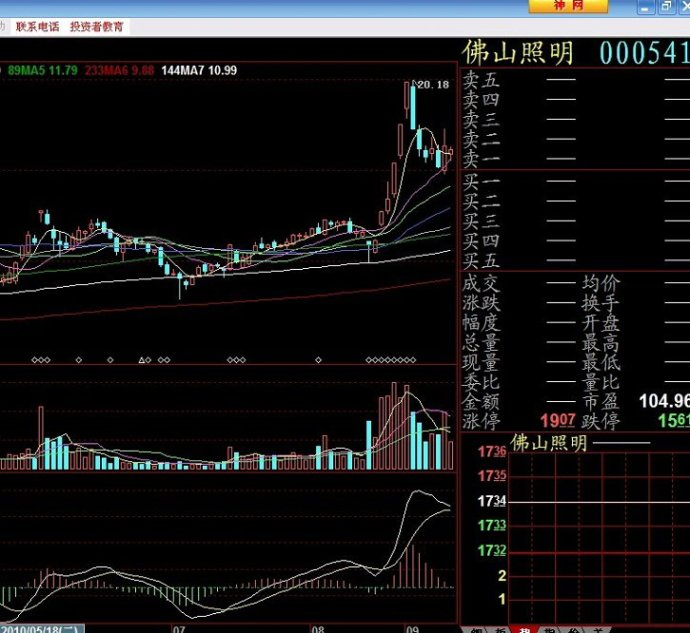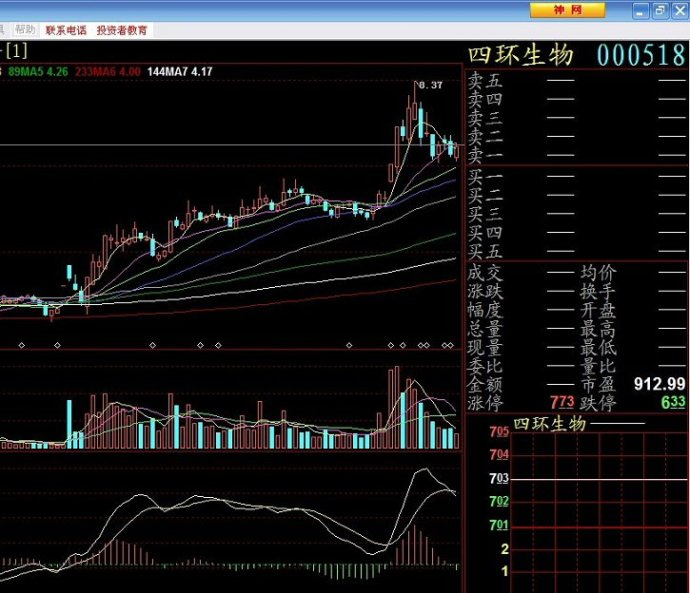N 1 500 5
N1 1 500 10
N2 1 500 20
N3 1 500 30
A1:=MA(CLOSE,N);
A2:=MA(CLOSE,N1);
A3:=MA(CLOSE,N2);
A4:=MA(CLOSE,N3);
CLOSE>A1 AND A1>A2 AND A2>A3 AND A3>A4 AND CLOSE>OPEN;
A1赋值:收盘价的N日简单移动平均
A2赋值:收盘价的N1日简单移动平均
A3赋值:收盘价的N2日简单移动平均
A4赋值:收盘价的N3日简单移动平均

{DDE均线多头选股公式}
JUJ:=(HIGH+LOW+CLOSE)/3;
QUJ0:=VOL/IF(HIGH=LOW,4,HIGH-LOW);
QUJ1:=IF(CAPITAL=0,QUJ0*(JUJ-MIN(CLOSE,OPEN)),QUJ0*IF(HIGH=LOW,1,(MIN(OPEN,CLOSE)-LOW)));
QUJ2:=IF(CAPITAL=0,QUJ0*(MIN(OPEN,CLOSE)-LOW),QUJ0*IF(HIGH=LOW,1,(JUJ-MIN(CLOSE,OPEN))));
QUJ3:=IF(CAPITAL=0,QUJ0*(HIGH-MAX(OPEN,CLOSE)),QUJ0*IF(HIGH=LOW,1,(HIGH-MAX(OPEN,CLOSE))));
QUJ4:=IF(CAPITAL=0,QUJ0*(MAX(CLOSE,OPEN)-JUJ),QUJ0*IF(HIGH=LOW,1,(MAX(CLOSE,OPEN)-JUJ)));
QUJ5:=QUJ1+QUJ2;
QUJ6:=QUJ3+QUJ4;
DD:=(QUJ5-QUJ6)/(QUJ5+QUJ6)*100;
DDZ:=DD*17;
DDX:=((QUJ1+QUJ2)-(QUJ3+QUJ4))/IF(CAPITAL=0,1000000,10000);
DX:=MA(DDX,5)*20;
DDX1:=SMA(DX,3,1);
DDX2:=SMA(DDX1,3,1);
DDX3:=EMA(DDX2,5);
DDY:=((QUJ2+QUJ4)-(QUJ1+QUJ3))/IF(CAPITAL=0,1000000,10000);
DY:=MA(DDY,5)*22;
DDY1:=SMA(DY,3,1);
DDY2:=SMA(DDY1,3,1);
DDY3:=EMA(DDY2,5);
CC:=ABS(REF(CLOSE,1)-CLOSE)/CLOSE*100;
JJ:=CC>0 AND CC<3;
AA:=DDX1>DDX2 AND DDX2>DDX3 AND DDX1>DDX3;
BB:=DDY1>DDY2 AND DDY2>DDY3 AND DDY1>DDY3;
HH:=AA>0 AND BB>0 AND DDZ>300 AND JJ>0;
HH>0;

A1:=MA(CLOSE,5);
A2:=MA(CLOSE,10);
A3:=MA(CLOSE,20);
A4:=MA(CLOSE,30);
CLOSE>A1 AND A1>A2 AND A2>A3 AND A3>A4 AND CLOSE>OPEN;

MA5:=MA(C,5);MA10:=MA(C,10);
A1:=MA5>=REF(MA5,1) AND MA5>=MA10;
A2:=C
A3:=BETWEEN(C,MA10,MA5*1.01);
A1 AND A2 AND A3;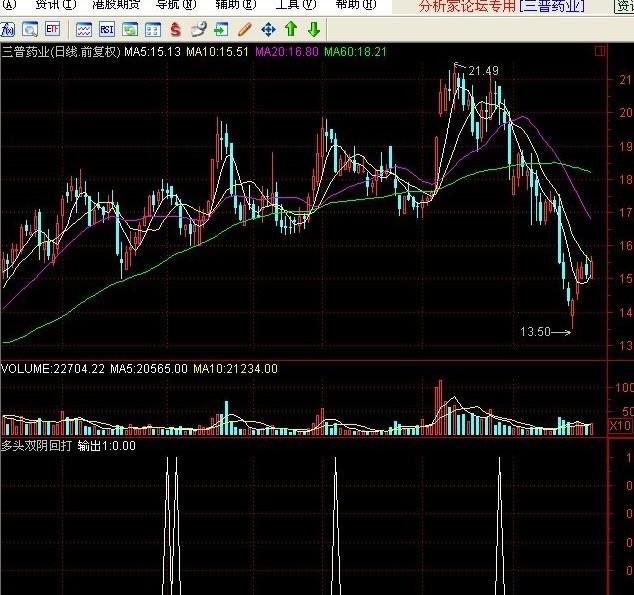MA5:=MA(CLOSE,5);
MA10:=MA(CLOSE,10);
MA20:=MA(CLOSE,20);
MA30:=MA(CLOSE,30);
A1:=MA5>MA10 AND MA10>MA20 AND MA20>MA30 AND ALL((MA5>MA10 AND MA10>MA20 AND MA20>MA30),D);
A2:=((MA5-MA10)/MA5)*100
A3:=A1 AND A2;
FILTER(A3,5);